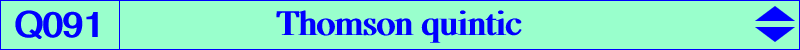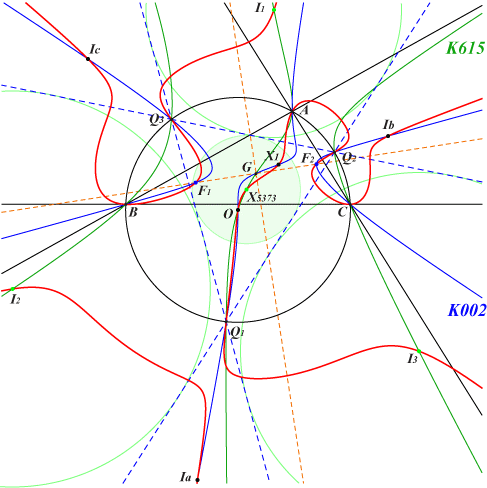too complicated to be written here. Click on the link to download a text file.X(1), X(3), X(5373) Q1, Q2, Q3 vertices of the Thomson triangle excenters I1, I2, I3 excenters of the Thomson triangle F1, F2 real foci of the Steiner inellipse infinite points of the McCay cubicThe Thomson triangle is formed by the intersections (apart A, B, C) of the Thomson cubic and the circumcircle. Q091 is the locus of M such that M and its two isogonal conjugates in the triangles ABC and Q1Q2Q3 are collinear. Q091 is also the locus of P such that there is a nodal cubic passing through the vertices of the triangles ABC, Q1Q2Q3 and whose tangents at its node P are perpendicular. See K626 for example. K258 is the locus of points whose polar conic in Q091 is a rectangular hyperbola. In other words, the cubic is the Laplacian of the quintic.